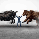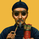3787 views
Invite-only script

Access to this script is restricted to users authorized by the author and usually requires payment. You can add it to your favorites, but you will only be able to use it after requesting permission and obtaining it from its author. Contact Marketzone for more information, or follow the author's instructions below.

TradingView does not suggest paying for a script and using it until you 100% trust its author and understand how the script works. In many cases you can find a good open-source alternative for free in our Public Library.

Want to use this script on a chart?//@version=2

strategy(title = "OCC+Supertrend", shorttitle = "OCC+Supertrend", overlay = true, pyramiding = 0, default_qty_type = strategy.percent_of_equity, default_qty_value = 10)

// === INPUTS ===
useRes = input(defval = true, title = "Use Alternate Resolution? ( recommended )")
stratRes = input(defval = "60", title = "Set Resolution ( should not be lower than chart )", type = resolution)
useMA = input(defval = true, title = "Use MA? ( otherwise use simple Open/Close data )")
basisType = input(defval = "DEMA", title = "MA Type: SMA, EMA, DEMA, TEMA, WMA, VWMA, SMMA, HullMA, LSMA, ALMA ( case sensitive )", type = string)
basisLen = input(defval = 14, title = "MA Period", minval = 1)
offsetSigma = input(defval = 6, title = "Offset for LSMA / Sigma for ALMA", minval = 0)
offsetALMA = input(defval = 0.85, title = "Offset for ALMA", minval = 0, step = 0.01)

// === /SUPERTREND/====

Factor=input(2, minval=1,maxval = 100)
Pd=input(10, minval=1,maxval = 100)
// === /SUPERTREND/====

// === /INPUTS ===

// === BASE FUNCTIONS ===
// Returns MA input selection variant, default to SMA if blank or typo.
variant(type, src, len, offSig, offALMA) =>
v1 = sma(src, len) // Simple
v2 = ema(src, len) // Exponential
v3 = 2 * v2 - ema(v2, len) // Double Exponential
v4 = 3 * (v2 - ema(v2, len)) + ema(ema(v2, len), len) // Triple Exponential
v5 = wma(src, len) // Weighted
v6 = vwma(src, len) // Volume Weighted
v7 = na(v5) ? sma(src, len) : (v5 * (len - 1) + src) / len // Smoothed
v8 = wma(2 * wma(src, len / 2) - wma(src, len), round(sqrt(len))) // Hull
v9 = linreg(src, len, offSig) // Least Squares
v10 = alma(src, len, offALMA, offSig) // Arnaud Legoux
type=="EMA"?v2 : type=="DEMA"?v3 : type=="TEMA"?v4 : type=="WMA"?v5 : type=="VWMA"?v6 : type=="SMMA"?v7 : type=="HullMA"?v8 : type=="LSMA"?v9 : type=="ALMA"?v10 : v1
// security wrapper for repeat calls
reso(exp, use, res) => use ? security(tickerid, res, exp) : exp
// === /BASE FUNCTIONS ===
// === /SUPERTREND/====

Up=hl2-(Factor*atr(Pd))
Dn=hl2+(Factor*atr(Pd))

TrendUp=close>TrendUp? max(Up,TrendUp) : Up
TrendDown=close<TrendDown? min(Dn,TrendDown) : Dn

Trend = close > TrendDown ? 1: close< TrendUp? -1: nz(Trend,1)
Tsl = Trend==1? TrendUp: TrendDown

linecolor = Trend == 1 ? green : red

plot(Tsl, color = linecolor , style = line , linewidth = 2,title = "SuperTrend")

// plotshape(cross(close,Tsl) and close>Tsl , "Up Arrow", shape.triangleup,location.belowbar,green,0,0)
// plotshape(cross(Tsl,close) and close<Tsl , "Down Arrow", shape.triangledown , location.abovebar, red,0,0)
//plot(Trend==1 and Trend==-1,color = linecolor, style = circles, linewidth = 3,title="Trend")

// plotarrow(Trend == 1 and Trend == -1 ? Trend : na, title="Up Entry Arrow", colorup=lime, maxheight=60, minheight=50, transp=0)
// plotarrow(Trend == -1 and Trend == 1 ? Trend : na, title="Down Entry Arrow", colordown=red, maxheight=60, minheight=50, transp=0)

// === /SUPERTREND/====

// === SERIES SETUP ===
// open/close
closeSeries = useMA ? reso(variant(basisType, close, basisLen, offsetSigma, offsetALMA), useRes, stratRes) : reso(close, useRes, stratRes)
openSeries = useMA ? reso(variant(basisType, open, basisLen, offsetSigma, offsetALMA), useRes, stratRes) : reso(open, useRes, stratRes)
trendState = closeSeries > openSeries ? true : closeSeries < openSeries ? false : trendState
// === /SERIES ===

// === PLOTTING ===
// barcolor(color = closeSeries > openSeries ? #006600 : #990000, title = "Bar Colours")
// channel outline
closePlot = plot(closeSeries, title = "Close Line", color = #009900, linewidth = 2, style = line, transp = 90)
openPlot = plot(openSeries, title = "Open Line", color = #CC0000, linewidth = 2, style = line, transp = 90)
// channel fill
closePlotU = plot(trendState ? closeSeries : na, transp = 100, editable = false)
openPlotU = plot(trendState ? openSeries : na, transp = 100, editable = false)
closePlotD = plot(trendState ? na : closeSeries, transp = 100, editable = false)
openPlotD = plot(trendState ? na : openSeries, transp = 100, editable = false)
fill(openPlotU, closePlotU, title = "Up Trend Fill", color = #009900, transp = 40)
fill(openPlotD, closePlotD, title = "Down Trend Fill", color = #CC0000, transp = 40)
// === /PLOTTING ===

// === STRATEGY ===
// conditions
longCond1 = (crossover(closeSeries, 1.00025*openSeries) and close>Tsl)
longCond2 = ((closeSeries> 1.00025*openSeries) and crossover(close,Tsl))
longCond3 = (close>Tsl and crossover(closeSeries, 1.00025*openSeries))
longCond4 = (crossover(closeSeries, 1.00025*openSeries) and close>Tsl)
shortCond1 = (crossover(openSeries, 1.00025*closeSeries) and Tsl<close)
shortCond2 = ((closeSeries< 1.00025*openSeries) and crossover(Tsl,close))
shortCond3 = (close<Tsl and crossover(openSeries, 1.00025*closeSeries))
shortCond4 = (crossover(openSeries, 1.00025*closeSeries) and close<Tsl)
longCond = longCond1 or longCond2 or longCond3 or longCond4
shortCond = shortCond1 or shortCond2 or shortCond3 or shortCond4
longExit = crossover(openSeries, closeSeries)
shortExit = crossover(closeSeries, openSeries)

// entries and base exit
strategy.entry("long", strategy.long, when = longCond)
strategy.entry("short", strategy.short, when = shortCond)

// not sure needed, but just incase..
// strategy.close("Long", when = longExit)
// strategy.close("Short", when = shortExit)
// === /STRATEGY ===